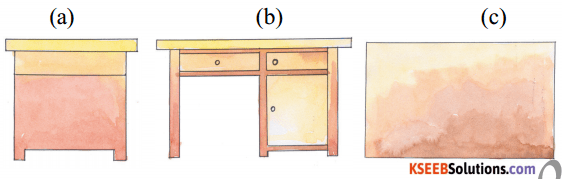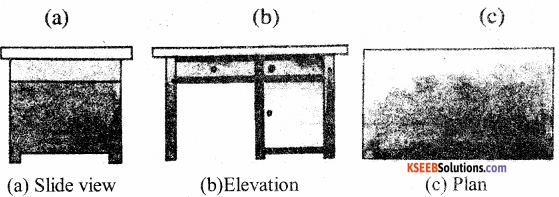# KSEEB Solutions for Class 5 Maths Chapter 9 Three Dimensional Figures

Students can Download Maths Chapter 9 Three Dimensional Figures Questions and Answers, Summary, Notes Pdf, KSEEB Solutions for Class 5 Maths helps you to revise the complete Karnataka State Board Syllabus and score more marks in your examinations.

## Karnataka State Syllabus Class 5 Maths Chapter 9 Three Dimensional Figures

### KSEEB Class 5 Maths Three Dimensional Figures Ex 9.1

Question a.
What is the shape of the faces of a cube?
Square

Question b.
How many circular faces are there in a cylinder?
TwoQuestion c.
Mention the number of plane surfaces and curved surfaces in a cone.
One, OneII. Observe the following table. Against each shape of solid, some pictures are given. Put (✓) against the figure which is an example of that shape and put (✗) against the figure which is not an example of that shape.III. A robot is constructed using different solid shapes. Identify the shapes and write the number of times it is used.Cube – 2, Cuboid -1, Cylinder – 3, Cone – 2IV. Different views of a table are given below. Name themV. 1. Draw diagrams of cube, cylinder and cone with different measurements in 2D.
Do it your self

2. Draw diagrams of your school showing elevation, side view and plan.
Do it your self

3. Draw diagrams of different objects in your house to show elevation, side view and plan.
Do it your selferror: Content is protected !!